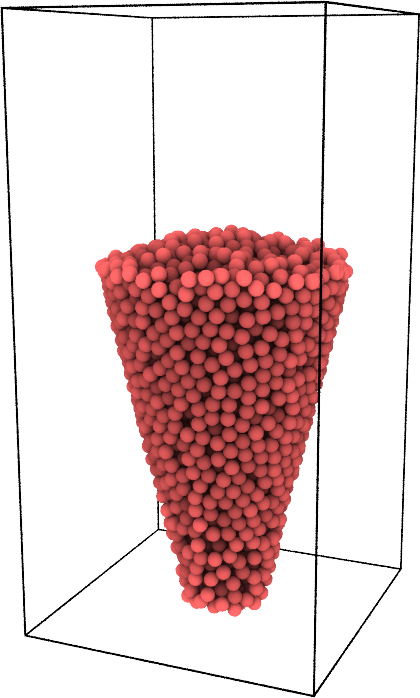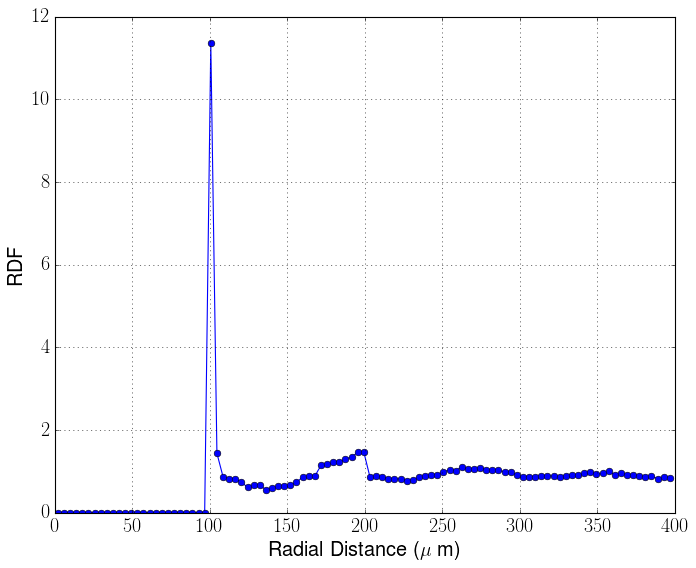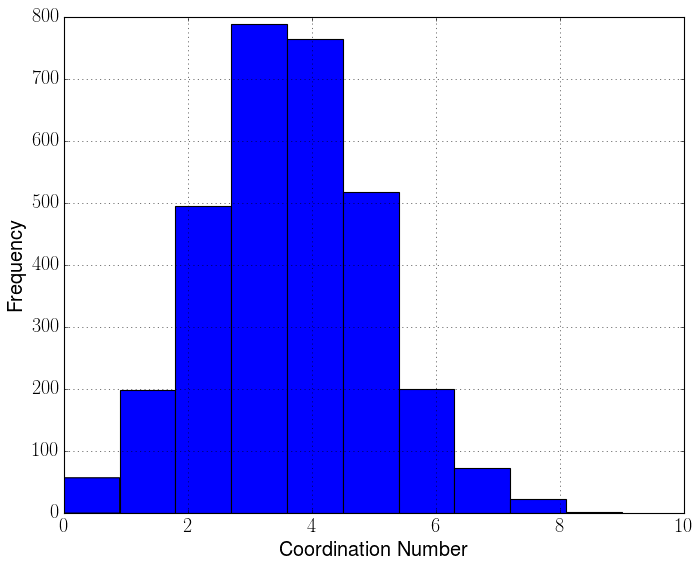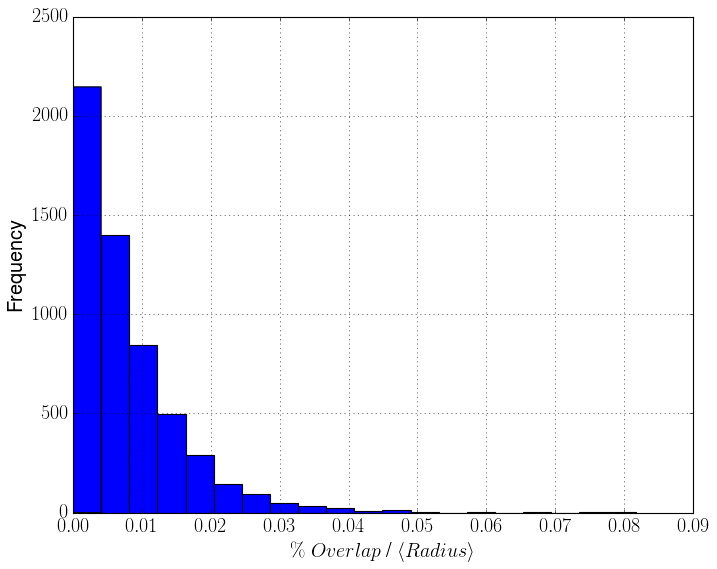# Strcutural Analysis¶

In this tutorial, a PyGran System class is created from a LIGGGHTS trajectory dump file. This class is then used to plot different spatial/structural variables.

In :
# Import PyGran + matplotlib modules
from PyGran import analysis
import matplotlib.pylab as plt

# Create a granular object from a LIGGGHTS dump file
Sys = analysis.System(Particles='traj*.dump')

Out:The code below constructs the RDF for the last frame in the system and then plots its using pylab.

In :
# Go to last frame
Sys.goto(-1)

# change unit system to micro
Sys.units('micro')

# Compute the radial distribution function
g, r, _ = Sys.Particles.rdf()

# Plot rdf vs radial distance
plt.plot(r, g, 'o-')

Constructing a cube of length 1579.55825864 and a circumscribed sphere of radius 394.889564659
Resolution chosen is 3.94889564659# Overlap Analysis¶

The code below constructs a histogram of all the particle-particle overlaps for the last frame in the system.

In :
# Construct a class for nearest neighbor searching
Neigh = analysis.equilibrium.Neighbors(Sys.Particles)

# Extract coordination number for all particles
coon = Neigh.coon()

# Construct + plot histogram of coon over 10 bins
plt.hist(coon)In :
# Extract the overlaps for all particles
overlaps = Neigh.overlaps[:,0]